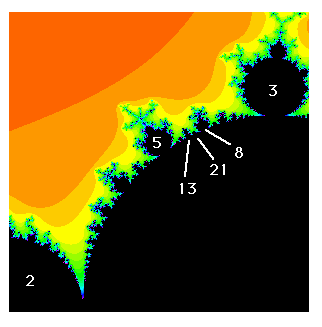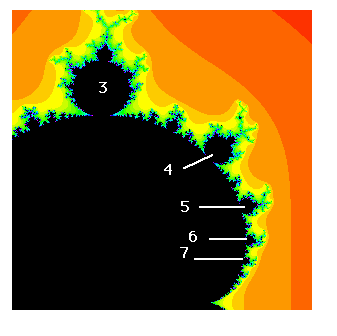## 7 The Fibonacci Sequence

The ideas in the previous section allow us to show the presence of the Fibonacci sequence in the Mandelbrot set. Forget for the moment about the rotation numbers and concentrate only on the periods of the bulbs (the denominators). Call the cusp of the main cardioid the ``period 1 bulb.'' Now the largest bulb between the period 1 and period 2 bulb is the period 3 bulb, either at the top or the bottom of the Mandelbrot set. The largest bulb between period 2 and 3 is period 5. And the largest bulb between 5 and 3 is 8, and so forth. The sequence generated (1, 2, 3, 5, 8, 13,...) is, of course, essentially the Fibonacci sequence. See Figure 11.Figure 11. The Fibonacci sequence: 1, 2, 3, 5, 8, 13, ...

There are many interesting sequences to be found in the Mandelbrot set. As before, the largest bulb between periods 1 and 2 is period 3. Then the largest between period 1 and 3 is period 4. The largest between 1 and 4 is 5, and so forth. The resulting sequence is 1, 2, 3, 4, 5, 6,.... I think that this sequence is destined to become very important in mathematics. In an effort to have my name attached to something, I call this sequence the Devaney sequence. I hope you will call it by its proper name too if you ever encounter this sequence.Figure 12. The Devaney Sequence: 1, 2, 3, 4, 5, 6,...8 Summary (Next Section)Fractal Geometry of the Mandelbrot Set (Cover Page)6 How to Add (Previous Section)